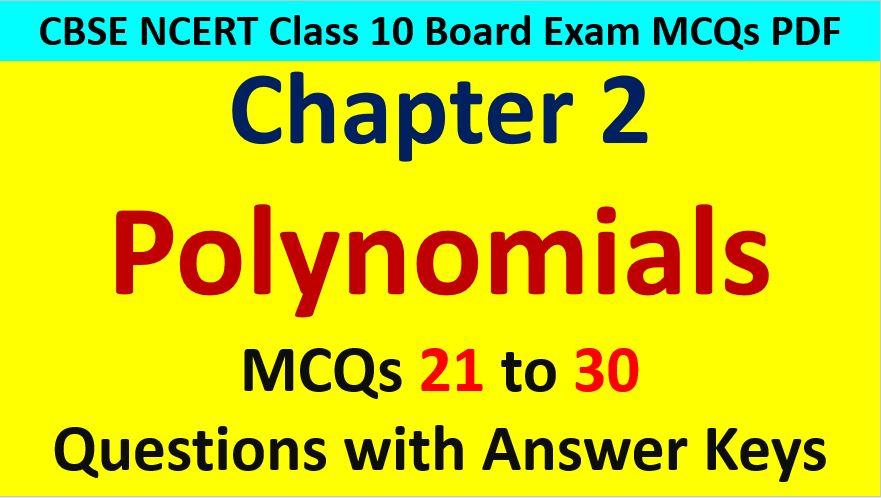Friday, October 22, 2021
Home > CBSE Class 10 > Important MCQ Questions for Class 10 Maths Chapter 2 Polynomials

# Important MCQ Questions for Class 10 Maths Chapter 2 PolynomialsHi students, Welcome to AMBiPi (Amans Maths Blogs). In this article, you will get Important MCQ Questions for Class 10 Maths Chapter 2 Polynomials. You can download this PDF and save it in your mobile device or laptop etc.

Important MCQ Questions of Polynomials Class 10 Question No 21:

What is the degree of the polynomial y3 – 2y2 – √y + 1/2?

Option A : 1/2

Option B : 2

Option C : 3

Option D : 3/2

Option C : 3

Important MCQ Questions of Polynomials Class 10 Question No 22:

If the sum and product of zeroes of a polynomial is 2 and -15 respectively, then the polynomial is

Option A : x2 – 2x + 15

Option B : x2 – 2x – 15

Option C : x2 + 2x – 15

Option D : x2 + 2x + 15

Option B : x2 – 2x – 15

Important MCQ Questions of Polynomials Class 10 Question No 23:

If one zero of a cubic polynomial ax3 + bx2 + cx + d is 0, then the value of the product of other two zeroes is

Option A : -c/a

Option B : c/a

Option C : 0

Option D : -b/a

Option B : c/a

Important MCQ Questions of Polynomials Class 10 Question No 24:

If a polynomial x3 + 4x2 – px + 8 is divisible by (x – 2), then the value of p is

Option A : 0

Option B : 3

Option C : 5

Option D : 16

Option D : 16

Important MCQ Questions of Polynomials Class 10 Question No 25:

If x = √(7 + 4√3), then the value of x + 1/x is

Option A : 4

Option B : 3

Option C : 2

Option D : 6

Option A : 4

Important MCQ Questions of Polynomials Class 10 Question No 26:

If the zeroes of a polynomial f(x) = 2x2 – 7x + 3 are p and q, then find the value of p2 + q2.

Option A : 7/2

Option B : 49/4

Option C : 37/4

Option D : 7

Option C : 37/4

Important MCQ Questions of Polynomials Class 10 Question No 27:

If the sum of any two zeroes of a cubic polynomial f(x) = x3 – px2 + qx – r, then

Option A : p + q = r

Option B : p – q = r

Option C : p/q = r

Option D : pq = r

Option D : pq = r

Important MCQ Questions of Polynomials Class 10 Question No 28:

If the zeroes of a polynomial f(x) = 2x2 + 6x – 6 are α and β, then

Option A : α + β = αβ

Option B : α + β > αβ

Option C : α + β < αβ

Option D : α + β + αβ = 0

Option A : α + β = αβ

Important MCQ Questions of Polynomials Class 10 Question No 29:

Which of the following is not a polynomial?

Option A : 2x/3 + 1

Option B : 2 – x2 + √3x

Option C : 1/(x – 1)

Option D : x3

Option D : It is not the graph of quadratic polynomial.

Important MCQ Questions of Polynomials Class 10 Question No 30:

HCF of 6x4 and 12xy is

Option A : 6x2y

Option B : 6x

Option C : 6y

Option D : 6xy